# How to Find Arbitrarily Large Fibonacci Numbers: A "C" Program

Knoji reviews products and up-and-coming brands we think you'll love. In certain cases, we may receive a commission from brands mentioned in our guides. Learn more.
Fibonacci sequence is a famous integer sequence in mathematics. The Fibonacci numbers appearing in the sequence rapidly increase with n so that we need to implement special technique for determining them. This article describes how to find both positive a

Fibonacci sequence is a very famous integer sequence in mathematics. The entries of the sequence are called Fibonacci numbers which are intimately related with the golden ratio. The sequence is an infinite and diverging sequence and so it is important to know a particular entry of the sequence, say nth Fibonacci number. But the problem is that the Fibonacci numbers increase very fast with increasing value of n and so we need to implement special technique for determining large Fibonacci numbers. In this article I will discuss how to find arbitrarily large Fibonacci numbers using a C program. Let us first know few basic properties of the numbers.

The Fibonacci Numbers

In the Fibonacci sequence each number is determined by the sum of the previous two with the convention that the first two (zeroth and first) are 0 and 1. Thus the sequence looks like follows.

0, 1, 1, 2, 3, 5, 8, 13, 21, 34, 55, 89, 144, 233, 377 …

Let us denote the nth  Fibonacci number by F(n) so that F(0) = 0, F(1) = 1 and F(n) = F(n-1) + F(n-2). Using the last recurrence relation we can also define negative Fibonacci numbers denoted by F(-n). All these properties in mathematical notation are shown below.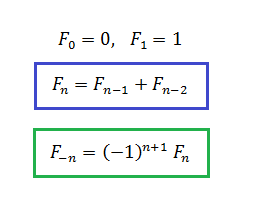Finding nth Fibonacci Number

Using the last two recurrence relations we can easily generate the sequence up to n terms using a computer program but the problem is, as easily seen, the numbers increase rapidly with n. Since all standard programming languages use 32bit arithmetic for integers the maximum value that can be stored in a variable is 2^32 = 4294967295 (considering ‘unsigned’ data type in C). So if used normally we will not be able to find a Fibonacci number greater than this maximum value. To overcome this limitation we have to use arrays.

The C Program

The C code provided below takes the value of n from user as input and prints the nth Fibonacci number.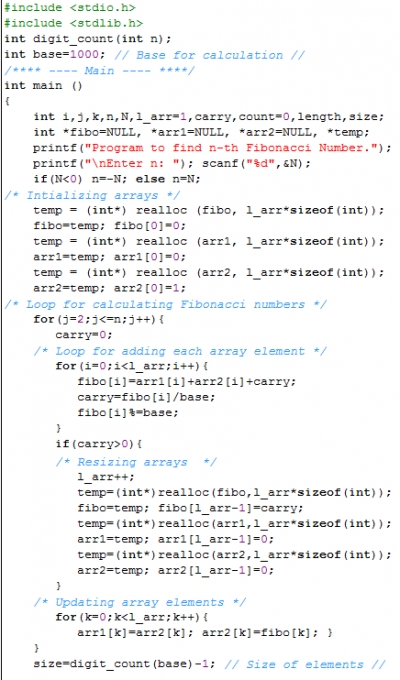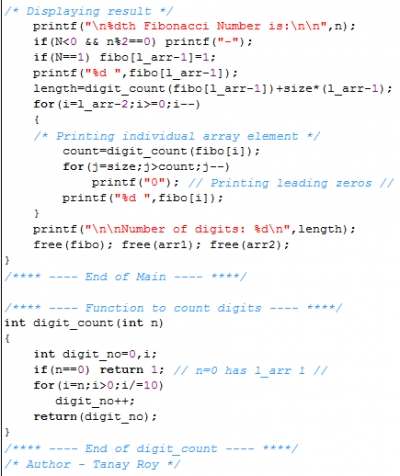The input can be positive or negative integers. Here, I have used base 1000 for calculations, which is suitable for handling large numbers in C. The command “realloc” is used for increasing the size of the array whenever the number of digits stored in an array element becomes greater than 3 (because of 3 zeros in the base used). For negative values of n the sign of the output is determined just before displaying the result. The function “digit_count” is called for counting number of digits in the final answer.

Since the algorithm automatically adjusts the size of array, any Fibonacci number of arbitrary size can be determined using this code unless no memory remains available for the calculation and storing numbers (for example the 123456th Fibonacci number, which contains 25801 digits, can be easily found within seconds). A typical output is shown below.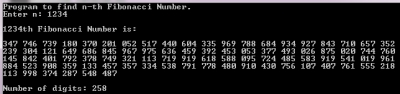Thus we have learned how to find arbitrarily large Fibonacci numbers using the C program.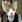thestickman
0
Posted on Jun 1, 2012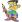roytanay
0
Posted on May 31, 2012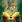deepa venkitesh
0
Posted on May 31, 2012